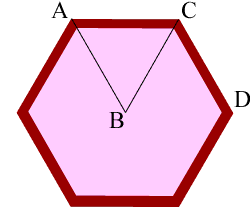Quandaries and Queries My husband is building a six sided wood circle and would like to know the angle to cut the pieces. thank you betty Hi Betty, I think I know what your husband is doing, he is making a hexagon.Since I am not sure how he is cutting this out I don't know what angles he needs. I have marked the triangle ABC and the hexagon is composed of 6 of these triangles. Since the circle is divided into 6 equal parts by these triangles, the angle at the center, angle ABC, is 360 degrees divided by 6 which is 60 degrees. Since the sides of the triangle AB and BC are equal, the angles CAB and BCA are equal. The sum of the angles in any triangle is 180 degrees and hence angle CAB is 60 degrees and angle BCA is 60 degrees. If he is making the hexagon by cutting around the outside then he needs to know the angle DCA. This is twice the angle BCA and hence it is 120 degrees. Penny Go to Math Central